# AP Board 7th Class Maths Solutions Chapter 5 Triangles Ex 5.4

SCERT AP 7th Class Maths Solutions Pdf Chapter 5 Triangles Ex 5.4 Textbook Exercise Questions and Answers.

## AP State Syllabus 7th Class Maths Solutions 5th Lesson Triangles Exercise 5.4

Question 1.
Two sides of a triangle are 5cm and 4cm respectively. Write any three possible measurements that suit the third side.
Given two sides of a triangle are 5 cm and 4 cm.
We know that, in triangle sum of lengths of any two sides is always greater than the third side, and in the triangle difference of lengths of any two sides is always less than the third side.
So, 5 cm, 4 cm, 2 cm
5 cm, 4 cm, 3 cm
5 cm, 4 cm, 4 cm
5 cm, 4 cm, 5 cm
5 cm, 4 cm, 6 cm
5 cm, 4 cm, 7 cm
Measurements that suit for third side are 2 cm, 3 cm, 4 cm, 5 cm, 6 cm, 7 cm.

Question 2.
The lengths of line segments are 3 cm, 5 cm, 6 cm and 9 cm.
(i) From the above measurements which group of the line segments can form a triangle.
3 cm, 5 cm, 6 cm and 5 cm, 6 cm, 9 cm groups form triangle.
Because, sum of any two sides is greater than the third side in this group.

(ii) Which group of line segments cannot form a triangle, give the reason?
We know that, sum of the lengths of any two sides is always greater than the third side.
3 cm, 6 cm, 9 cm does not form a triangle.
Because sum of 3 cm and 6 cm is not greater than 9 cm.Question 3.
Find the value of ‘x’ in the following triangles :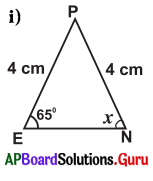Given, in ∆PEN, PE = 4 cm, PN = 4 cm ∠E = 65°, ∠N = x°
Two sides are equal in length.
So, ∆PEN is an isosceles triangle.

In isosceles triangle, the angles which are opposite to equal sides are also equal.
So, ∠N = ∠E
∴ x = 65°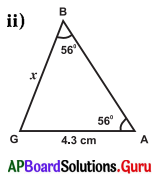Given, in ∆BGA,
∠B = 56°, ∠G = x, ∠A = 56° and BG = x cm, GA = 4.3 cm
We know that, sum of angles of a triangle is 180°.
In ∆BGA, ∠B + ∠G + ∠A = 180°
⇒ 56° + x + 56° = 180°
⇒ 112° + x = 180° :
⇒ 112° + x – 112° =180°- 112°
∴ x = 68°

Two angles are equal in ∆BGA.
So, ∆BGA is an isosceles triangle.
In isosceles triangle the sides which are opposite to equal angles are also equal.
So, BG = GA
∴ x = 4.3 cm

Question 4.
∆ABC is an isosceles triangle in which AB = AC. If ∠A = 80°, then find ∠B and ∠C.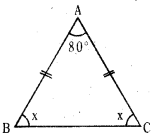In isosceles triangle the angles which are opposite to equal sides are also equal.
So, ∠B = ∠C = x°
In ∆ABC, we know
∠A + ∠B + ∠C — 180°
⇒ 80° + x + x = 180°
⇒ 80° + 2x – 80° = 180° – 80°
⇒ 2x = 100°
⇒ $$\frac{2 x}{2}=\frac{100^{\circ}}{2}$$
∴ x = 50°
∴ ∠B = ∠C = 50°Question 5.
Find the values of ‘x’ in each of the below triangles.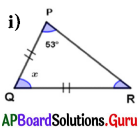In ∆PQR, PQ = QR and ∠P = 53°
∆PQR is an isosceles triangle.

In isosceles triangle, the angles which are opposite to equal sides are also equal.
So, ∠P = ∠R = 53°
In ∆PQR, we know
∠P + ∠Q + ∠R = 180°
⇒ 53° + x + 53° = 180°
⇒ 106° + x = 180°
⇒ 106° + x- 106° = 180°- 106°
∴ x = 74°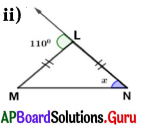In ∆LMN, LM = LN and exterior angle at L = 110° .
∆LMN is an isosceles triangle.
In isosceles triangle, the angles which are opposite to equal sides are also equal.

So, ∠M = ∠N = x° .
We know that an exterior angle of a triangle is equal to the sum of its opposite interior angles.
Exterior angle at
L = ∠M + ∠N = 110°
⇒ x + x = 110°
⇒ 2x = 110°
⇒ $$\frac{2 x}{2}=\frac{110}{2}$$
∴ x = 55°

Question 6.
Which of the following statements are true in the following diagram.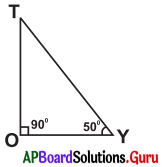(i) OY < OT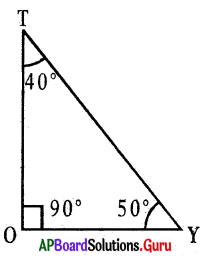In ∆TOY, ∠T + ∠O + ∠Y = 180°
⇒ ∠T + 90° + 50° = 180°
⇒ ∠T + 140° = 180°
⇒ ∠T + 140°- 140° = 180° – 140°
∴ ∠T – 40°
We know that side opposite to the largest angle is longest.
OY< OT – True

(ii) TY < TO